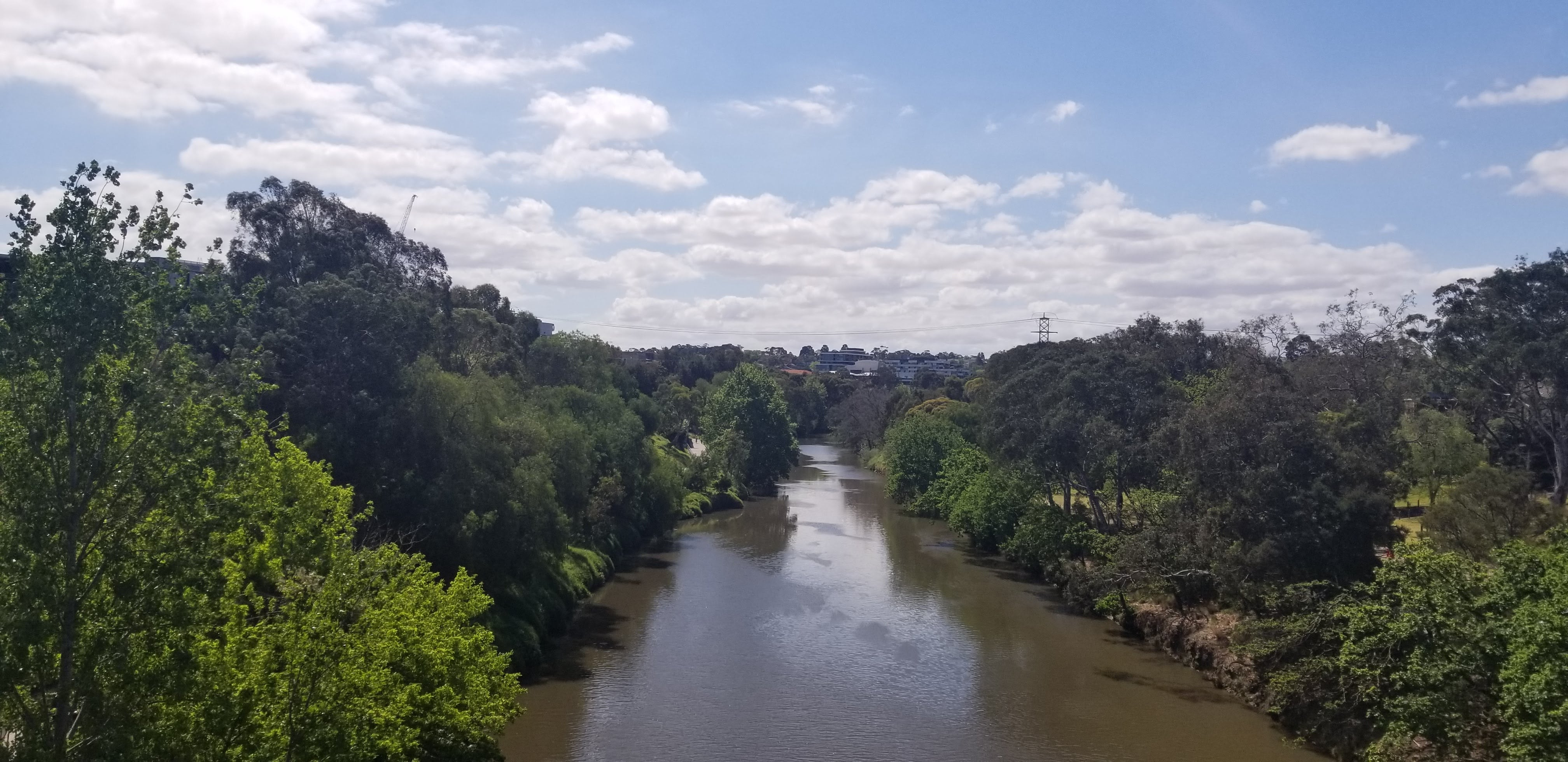# 180 degree southThe best journey answers the question that in the beginning you didn’t even think to ask

# BERT based sentence scenario detector

`# 模型构建model = Sequential([    Conv1D(filters=5, kernel_size=5, strides=1, padding='valid', input_shape=(768, 1), name="Convolution_Layer_1"),    AveragePooling1D(pool_size=5, strides=1, padding="valid", name="Pooling_Layer_1"),    Conv1D(filters=5, kernel_size=5, strides=1, padding='valid', name="Convolution_Layer_2"),    AveragePooling1D(pool_size=5, strides=1, padding="valid", name="Pooling_Layer_2"),    Flatten(name="Flatten_Layer"),    Dense(256, input_dim=3760, name="Dense_Layer_1"),    Activation('relu'),    Dropout(0.1),    Dense(32, input_dim=256, name="Dense_Layer_2"),    Activation('relu'),    Dropout(0.1),    Dense(11, input_dim=32, name="Dense_Layer_3"),    Activation('softmax'),])`

# 通用场景识别器

`# 模型构建model = Sequential([    Dense(32, input_dim=200),    Activation('relu'),    Dropout(0.1),    Dense(16, input_dim=32),    Activation('relu'),    Dense(9, input_dim=32),    Activation('softmax'),])`

` test loss:  0.09276254528926478 test accuracy:  0.9666666666666667`